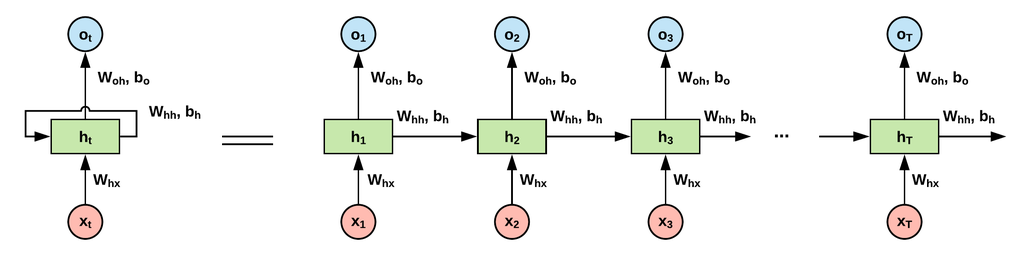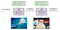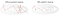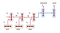# Introduction to RNN’s

Introduction

Recurrent Neural Networks (RNNs) were very popular models before the advent of Transformer based models. They showed great performances in many NLP tasks.

In order to understand RNNs, we will first see what problems they solve, how they work in theory and how the model is trained. We will also create a vanilla model in Pytorch.

What problem does RNN solve ?

In traditional neural networks e.g. convolutional neural networks, all the inputs are independent of each other and they primarily recognize the patterns in the data but in order to handle the sequential data, we need recurrent neural networks, making it easier to perform predictions on sequential data. Recurrent means that they perform the same task for every element in the sequence which is dependent on the computation of previous computations. The key component of the recurrent neural network is to remember/memorize data from the past sequence that it has seen and uses it to make predictions much more accurately.

RNNs are good for recognizing patterns in sequences of data:

• Time series
• Text data
• Genomes
• Spoken word

Theory

In this network, the information moves in only one direction, forward, from the input nodes, through the hidden nodes (if any) and to the output nodes. There are no cycles or loops in the network. — Wikipedia

Firstly, the recurrent network needs to be trained on a large dataset to make better predictions. To understand RNNs better, let’s go through a simplest example of generating the next word in a sequence using previously seen words in a sentence.

We first feed a single word to the network, the network makes a prediction and we use the prediction and the next word and feed it to the network in the next block. Here you can compare how the feed forward neural network is different from recurrent neural networks. For RNNs, we need to compute the previous state in order to compute the current state.http://www.easy-tensorflow.com/tf-tutorials/recurrent-neural-networks/vanilla-rnn-for-classification

Here xi are the input texts and since we cannot feed plain text to the network, we need to use embeddings to encode the words into vectors. (Other approaches like one-hot vector can also work but embeddings are the best choice. )

Training loop:

• Sample input text from the dataset
• Convert the input text into embeddings.
• Feed the input to the network which will perform complex computations on it using randomly initialized variables.
• Generates a prediction as an output
• Check how different is prediction with the original value
• Calculate the loss.
• Do a back propagation and adjust the variables.
• Repeat the above steps.
• Perform predictions on unseen/test dataset.

Mathematical Equations:

Equation 1: Information from previous timestamp in the sequence is propagated to the current word. Ht is calculated from the h(t-1) vector and the current vector. An activation function is also applied. You can also think of Ht as a memory vector. It stores all the information that might be helpful e.g. frequency of positive/negative words etc.

Equation 2: calculates the probability distribution and gives us the index of the highest probable next word. The softmax function produces a vector summing up to 1.

Equation 3: It’s a cross-entropy loss which will calculate the loss at a particular time stamp t. It is calculated based on the difference between predicted and actual word.https://www.topbots.com/exploring-lstm-tutorial-part-1-recurrent-neural-network-deep-learning/

Code in Pytorch

Shortcomings

There were alot of problems with vanilla RNNs, as we can see from the

• Memory is rewritten at each step
• Gradients tend to vanish or explode

As RNNs are prone to vanishing or exploding gradients, we will implement gradient clipping to prevent the gradient from “exploding” which means if the calculated gradient is larger than a certain threshold, we will scale it back to the threshold.Gradient descent with and without clipping. Source
• Difficult to capture long-term dependencies
• Difficult to train

Different variants of RNN’s

One to One: Feed Forward Neural Network

One to Many: e.g. Image Captioning image -> sequence of words

Many to One: e.g. Sentiment Classification (sequence of words -> sentiment)

Many to Many: e.g. Machine Translation (seq of words -> seq of words) or .g. Video classification on frame level

Example: Machine Translation

One use case of RNNs is machine translation, given an input sequence of words in a particular language, we would like to convert it into a different target language e.g. German (source) → English (Target). In this case, we need to have a complete sentence encoded before generating the output sentence in another language because the source sentence might have key information later in the sentence that is required by the first word of the target sentence.Source: http://cs224d.stanford.edu/lectures/CS224d-Lecture8.pdf

Conclusion

I hope you understand the core concept behind RNN’s, what problem it solves and how it works. Pytorch implementation gives an overview from the coding perspective. Although vanilla RNNs are not used much in industry and there are better variants ie. LSTM and GRU which we will cover in next posts.

References:

On the road to make some impact.

## More from Sami.

On the road to make some impact.